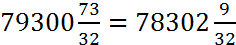# Multiplication of Fractions

Reduce the fractions to simple fractions. Then multiply the numerators together and multiply the denominators together. If the two numbers are mixed factions (a whole number and a fraction) then multiply the whole numbers together, multiply the fractions together, multiply each whole number by the fraction associated with the other whole number and add the four terms together.

Examples:

(1) Multiply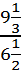by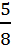of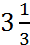of 7.

This can be expressed as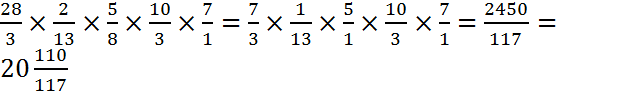.

(2) Multiply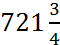by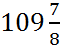.

The four terms are:

(a)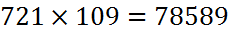(b)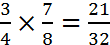(c)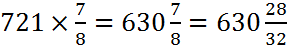(d)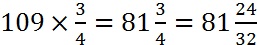Total: